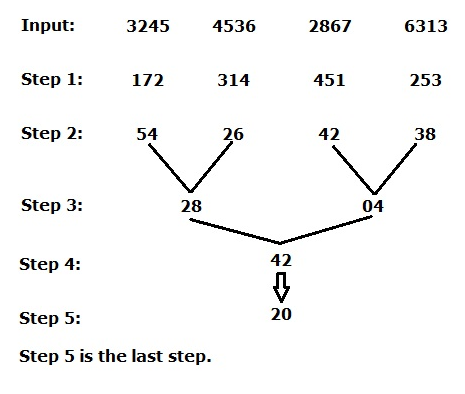# SBI Clerk Mains 2021 Reasoning Ability (Day-03)

Dear Aspirants, Our IBPS Guide team is providing new series of Reasoning Questions for SBI Clerk Mains 2021 so the aspirants can practice it on a daily basis. These questions are framed by our skilled experts after understanding your needs thoroughly. Aspirants can practice these new series questions daily to familiarize with the exact exam pattern and make your preparation effective.

Start Quiz

Coded inequality

Directions (1-3): In the following questions, the symbols %, \$, @, & and # are used with the following meanings as illustrated below. In each question below there are some statements followed by three/four conclusions numbered I, II, III and IV. Read the statement carefully and then decide which of the following conclusion logically follows disregarding commonly known facts.

‘A @ B’ means ‘A is greater than B’

‘A # B’ means ‘A is smaller than B’

‘A % B’ means ‘A is greater than or equal to B’

‘A \$ B’ means ‘A is less than or equal to B’

‘A & B’ means ‘A is equal to B’

1) Statement:
[email protected], L#F, L&O%P, A&D, S\$D,

Conclusions:

ii) S#F

A. only conclusion ii follows

B. None follows

C. only conclusion i and iii follows

D. All i, ii and iii follows

E. only conclusion ii and iii follows

#### 2) Statement: X%K, X%Y, U&Y, P#U, S%P, R#S

Conclusions:

i) K&U

ii) Y#S

iv) X&U

A. only conclusion ii follows

B. None follows

C. only conclusion i and iii follows

D. All i, ii and iii follows

E. Either iii or iv follows

3) Statement:
[email protected], M%P, [email protected], X&N, Z#X, A\$Z, B\$A

Conclusions:

i) P&X

ii) P%Z

iii) M#A

iv) B&P

A. only conclusion ii follows

B. None follows

C. only conclusion i and iii follows

D. All i, ii and iii follows

E. only conclusion ii and Either iii or iv follows

Coded direction sense

Direction (4-5): Read the following information carefully and answer the questions given below.

‘P @ Q’ means ‘P stands 5 metres to the North of Q’

‘P # Q’ means ‘P stands 5 metres to the South of Q’

‘P % Q’ means ‘P stands 5 metres to the East of Q’

‘P \$ Q’ means ‘P stands 5 metres to the West of Q’

4) In which direction is Z with respect to R from the given expression ‘[email protected]%[email protected][email protected]\$X#Y\$Z’?

A. Southwest

B. Northwest

C. Southeast

D. Northeast

E. North

5) In which direction is S with respect to J from the given expression ‘[email protected]
[email protected][email protected]@J’?

A. Southwest

B. Northwest

C. Southeast

D. Northeast

E. North

Input output

Directions (6-10): Study the following information carefully to answer the given questions.

A number arrangement machine when given an input line of numbers rearranges them following a particular rule in each step. The following is an illustration of input and rearrangement.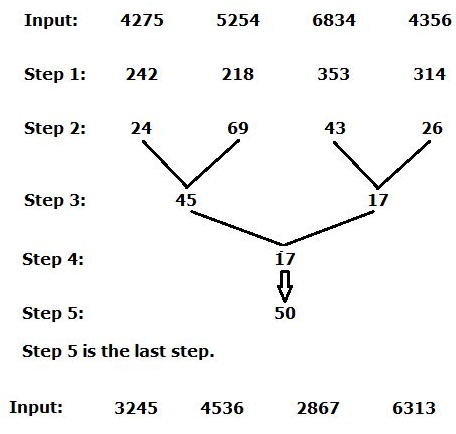6) What is the sum of numbers in Step 3?

A.42

B.62

C.32

D.112

E.None of these

7) How many numbers are between 2nd digit of 2nd number from theright end and 1st digits of 1st number from theleft endin step 2?

A.None

B.Two

C.Five

D.Seven

E.None of these

8) What is the difference between numbers in step 4 and step 5?

A.17

B.28

C.34

D.22

E.None of these

9) Which of the following number is obtained in the penultimate step?

A.28

B.42

C.04

D.20

E.None of these

10) Which of the following is the second highest number in step 2?

A.26

B.42

C.38

D.54

E.28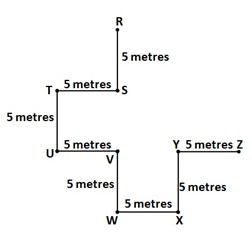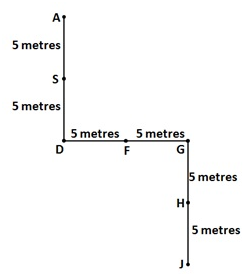Directions (6-10) :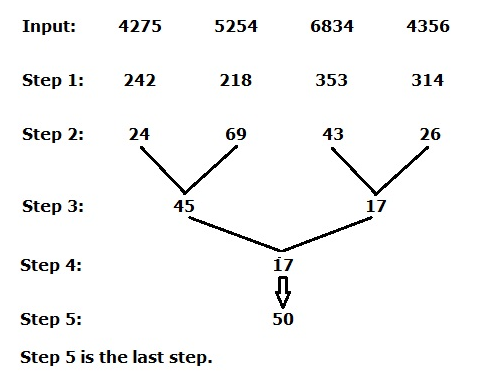Step 1: 1st and 4th digits in each number are changed to its cube value. 2nd and 3rd digit of each number is squared. Then sum them all numbers. 4275 as 64+125+4+49= 242 same methods for all the numbers in step 1.

Step 2: All the digits in the numbers are changed to its square value and sum all of the three values. Such as 242 as 4+16+4=24.

Step 3: Difference between1st and 3rdnumber with 2nd and 4thnumber respectively. Large numberis subtracted with small number.

Step 4: Interchange the position of the digits within thenumber then take the difference between the two numbers. 45 is changed to 54 and 17 change to 71. Then 71-54 = 17.

Step 5: Sum of the digits which is changed to its square value, 1+7 => 1+49 =50.